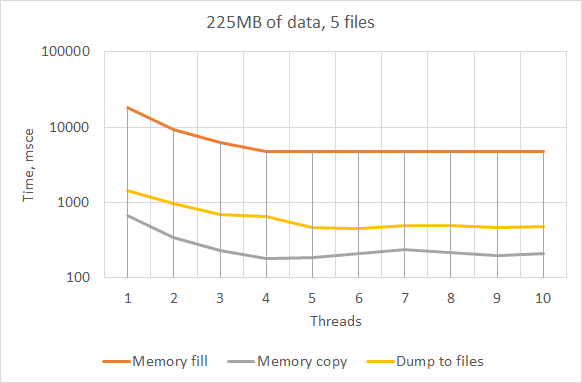The small test program shows how memory and file I/O operations can be accelerated using multiple threads.

# Scenario

The program uses

• X bytes of memory to allocate a huge block (255 MB by default)
• M files to dump some huge memory block

The running program

• allocates a first memory block (byte array) of X bytes
• fills it with random byte values
• allocates a second memory block
• copy the first array values into it
• write the array content by chunks of X/M bytes to M files

# Test program

The program doesn't use any concurrency control synchronizations because the processed memory chunks aren't intersected so there is no data race conditions.

``````#include <iostream>
#include <sstream>
#include <fstream>
#include <string>
#include <vector>
#include <ctime>
#include <chrono>

using namespace std;
using namespace std::chrono;

typedef char TestDataType;
TestDataType* test_data;
TestDataType* test_data_copy;

void fill_data(const size_t from, const size_t to)
{
for (size_t i = from; i < to; i++)
test_data[i] = static_cast<TestDataType>(rand());
}

void copy_data(const size_t from, const size_t to)
{
for (size_t i = from; i < to; i++)
test_data_copy[i] = test_data[i];
}

void save_mem_to_file(const string file_name, const size_t from, const size_t to)
{
ofstream out(file_name, ofstream::trunc);
out.write(&test_data[from], to - from);
out.close();
}

int main(int argc, char* argv[])
{
if (argc > 1)
int file_count = 10;
if (argc > 2)
file_count = atoi(argv);
size_t data_length = 255 * 1024 * 1024; // 255 MB by default
if (argc > 3)
data_length = atol(argv);
size_t batch_size = data_length / thread_count;
<< "Batch size: " << batch_size << " bytes" << endl
<< "Files count: " << file_count << endl;

try
{
srand(static_cast<unsigned int>(std::time(nullptr)));
// Fill memory (random values)
cout << "Starting memory fill test" << endl;
test_data = new TestDataType[data_length];
system_clock::time_point t11 = system_clock::now();
for (int i = 0; i < thread_count; i++)
{
size_t from = batch_size * i;
size_t to = from + batch_size - 1;
cout << "Thread " << i << ": " << from << " - " << to << endl;
}
th.join();
system_clock::time_point t12 = system_clock::now();
auto ms1 = std::chrono::duration_cast<std::chrono::milliseconds>(t12 - t11);
cout << "Memory fill finished" << endl;
cout << "Duration, msec: " << ms1.count() << endl;
// Copy memory
cout << "Starting memory copy test" << endl;
test_data_copy = new TestDataType[data_length];
system_clock::time_point t21 = system_clock::now();
for (int i = 0; i < thread_count; i++)
{
size_t from = batch_size * i;
size_t to = from + batch_size - 1;
cout << "Thread " << i << ": " << from << " - " << to << endl;
}
th.join();
system_clock::time_point t22 = system_clock::now();
auto ms2 = std::chrono::duration_cast<std::chrono::milliseconds>(t22 - t21);
cout << "Memory copy finished" << endl;
cout << "Duration, msec: " << ms2.count() << endl;
delete test_data_copy;
// Save to files
string file_name_base = "testdump";
system_clock::time_point t31 = system_clock::now();
size_t file_size = data_length / file_count;
int i = 0, j = 0;
while (i < file_count)
{
{
size_t from = file_size * j;
size_t to = from + file_size - 1;
stringstream file_name;
file_name << file_name_base << i << ".dmp";
cout << "Thread " << i << ", file " << file_name.str() << ": " << from << " - " << to << endl;
i++;
j++;
}
else
{
j = 0;
th.join();
}
}
th.join();
system_clock::time_point t32 = system_clock::now();
auto ms3 = std::chrono::duration_cast<std::chrono::milliseconds>(t32 - t31);
cout << "Memory writing finished" << endl;
cout << "Duration, msec: " << ms3.count() << endl;

cout << "Test finished" << endl;
delete test_data;
}
{
cout << "Insufficient memory. Error: " << e.what() << endl;
}
catch (std::exception& e)
{
cout << e.what() << endl;
}
catch (...)
{
cout << "Unexpected exception" << endl;
}
}
``````

# Results

Tests are performed on the development PC

• Intel i5-3470, 4 cores at 3.2 GHz, no hyper-threading
• 16 GB of RAM
• RAID-1 disk array with classic HDD 7200 tr/min
• Windows 10 Pro

The test program is compiled with Microsoft (R) C/C++ Optimizing Compiler Version 19.13.26132 for x86 (Visual Studio 2017 Community Edition).

Number of threads Memory fill, msec Memory copy, msec Dump to files, msec
1 18465 674 1448
2 9313 339 982
3 6245 230 692
4 4789 183 650
5 4788 185 465
6 4725 207 452
7 4748 238 488
8 4705 217 495
9 4776 199 462# Conclusions

It can be seen that a program manipulating multiple threads can accelerate not only CPU-used code execution (like random values generating) but simple I/O operation like memory copy or writing to multiple files.

As expected, using the number of threads exceeding the CPU core count doesn't have any effect. Try to test it in hyperthreading mode.